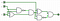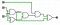# Deobfuscating code for fun and no profit round 2

If you missed part 1 you can check it out here.

Welcome back! I already did a bit of intro in the last article so we’ll skip that here. Basically we’re going to be looking at an obfuscated program and figuring out how it works — so let’s jump into the code! I’ve picked heathbar.c from the 1995 IOCCC. I’d love to reproduce the original program here but medium wraps the lines and it’s….even more unreadable. I recommend checking it out at the link I gave but here is a high level picture — this one is mostly about the ASCII art anyway.

So if you’re unaware what you’re looking at is an ASCII art representation of an Adder. It doesn’t look like some of the wikipedia examples but I simulated the circuit and it’s definitely an adder. This gives a clue about what the program does — it adds two numbers. Specifically, it adds two 16 bit unsigned integers by simulating a bunch of adders chained together.

If you’d like to run the program I was able to compile it on Ubuntu 16.04 with `cc -ansi -O -w --std=c89 heathbar.c -o heathbar` , after that run it with some numbers like so: `./heathbar 25 50` . Easy enough.

# Step 1: Unraveling the source

First thing I like to do is replace the defines — this program relies heavily on the defines for obfuscation so removing them will definitely help. After we do that, and clean up whitespace; the program looks like this:

`#include <stdio.h>int mAIn(int), atoi(char *), maIn = -1, ma1n, maiN;int main(int Ma1n, char **mAin){    if (Ma1n != 3)        return 0;maIn++, Ma1n--, mAin++;    maIn = atoi((Ma1n--, *mAin++));    ma1n = atoi((Ma1n--, *mAin++));mAIn(mAIn(mAIn(mAIn(mAIn(mAIn(mAIn(mAIn(mAIn(mAIn(mAIn(mAIn(mAIn(mAIn(mAIn(mAIn(Ma1n))))))))))))))));printf("%d\n", maiN);return 0;}int mAIn(int mAin){    static int main = -1;main++;return 0, maiN |= ((!!(maIn & 1 << main) || ((!!(ma1n & 1 << main) || mAin) && (!(!!(ma1n & 1 << main) && mAin))))            && (!(!!(maIn & 1 << main) && ((!!(ma1n & 1 << main) || mAin) && (!(!!(ma1n & 1 << main) && mAin)))))) << main,            (!!(ma1n & 1 << main) && mAin) || (!!(maIn & 1 << main) && ((!!(ma1n & 1 << main) || mAin) && (!(!!(ma1n & 1 << main) && mAin))));}`

Hm…better but still not great. We should replace some more of those “main” variables. In the main function (the real ‘main’) argc/argv have been changed so I’ll put those back. The atoi prototype I think is unnecessary (I compiled without it). the “mAIn” function which gets called repeatedly is our simulated adder so I changed that to “add”. maIn, and ma1n are our numbers being added so I’ll call them x and y. maiN is the variable holding our final result so I’ll call that result.

Here is what the first half looks like now:

`int add(int);int x = -1, y, result;int main(int argc, char **argv){    if (argc != 3)        return 0;    x++, argc--, argv++;    x = atoi((argc--, *argv++));    y = atoi((argc--, *argv++));add(add(add(add(add(add(add(add(add(add(add(add(add(add(add(add(argc))))))))))))))));    printf("%d\n", result);    return 0;}`

That was all just cleanup, we haven’t really removed any “real” obfuscation from the main function. Let’s do that next before we unravel the real beast.

# Step 2: Debofuscating the main function

The first notable thing that happens is probably the `x++, argc--, argv++` line — and in the line right after it we assign x to the result of that atoi call. Don’t need to bother incrementing it then…in fact we don’t need it initially set to -1 at all.

Next I’d focus on those atoi calls — looks pretty weird right? atoi doesn’t accept two arguments. Well, notice that the argument(s) are wrapped in brackets and they are separated by a comma. This is actually the comma operator at work — it will evaluate the argument on the left but the argument on the right is the only thing being returned. Essentially the result of *argv++ is what gets passed to atoi. Since we run this from the command line with two arguments it becomes apparent that this part is parsing our command line integer arguments.

So if the result of `argc--` is discarded is it worth it to do those operations? Kind of…it is passed to our add function but at that point it has a value of 0, this will never change so it’s perfectly fine to remove any `argc--` statements and just pass in 0 to the add function. We still need the `argv++` stuff though.

Now we can get into what the add function is actually doing, but just for reference here are the lines I changed:

`int x, y, result;int main(int argc, char **argv){    if (argc != 3)        return 0;    argv++;    x = atoi(*argv++);    y = atoi(*argv++);add(add(add(add(add(add(add(add(add(add(add(add(add(add(add(add(0))))))))))))))));`

# Step 3: Unraveling the add function

Here is the function we’ll be unraveling (note: the argument is argv because it had the same variable as main’s argv before the search/replace):

`int add(int argv){    static int main = -1;    main++;return 0, result |= ((!!(x & 1 << main) || ((!!(y & 1 << main) || argv) && (!(!!(y & 1 << main) && argv))))            && (!(!!(x & 1 << main) && ((!!(y & 1 << main) || argv) && (!(!!(y & 1 << main) && argv)))))) << main,            (!!(y & 1 << main) && argv) || (!!(x & 1 << main) && ((!!(y & 1 << main) || argv) && (!(!!(y & 1 << main) && argv))));}`

The first thing I notice is the return statement — it looks like it’s returning multiple stuff. It’s actually just the comma operator in action again. The first 0 is never returned, the second comma arg is not returned but it does modify the result variable, the third comma arg is actually the only one that is returned. This basically breaks up the super long statement into 2 different long statements.

The next thing to notice is stuff like `!!(x & 1 << main)` being repeated a lot. After some fun with printf I realized that this was selecting the nth bit from variable x (or y) and returning whether that bit was set each time the add function was called — the bit being selected increased sequentially. This is because the “main” variable is static so the value is persisted between function calls. Knowing this I’d like to rename “main” to “pos” (because it’s our position/index in the variable). We can also assign the result of `!!(x & 1 << main)` to a variable and save ourselves a lot of space. I’m going to call the vars `b1` and `b2` . I also renamed the `argv` being passed to the add function `carry` . This is just adder terminology and honestly I’m not 100% sure that’s what it is…but I’m like 98% sure. Worth noting that this means the value being returned by the add function is also the carry.

This cleans the function up into a slightly less insane version:

`int add(int carry){    static int pos = -1;    pos++;    int b1 = !!(x & 1 << pos);    int b2 = !!(y & 1 << pos);    result |= ((b1 || ((b2 || carry) && (!(b2 && carry)))) && (!(b1 && ((b2 || carry) && (!(b2 && carry)))))) << pos;    return (b2 && carry) || (b1 && ((b2 || carry) && (!(b2 &&  carry))));}`

That still isn’t perfect, and I have to say simplifying the expressions doesn’t help much (believe me — I tried). If you’re curious here are logic gate diagrams for the statements:This is: ((b1 || ((b2 || carry) && (!(b2 && carry)))) && (!(b1 && ((b2 || carry) && (!(b2 && carry))))))This is: (b2 && carry) || (b1 && ((b2 || carry) && (!(b2 && carry))))

So there isn’t much you can do with what we’re given, although if you’re interested in seeing what this program basically does in a conceptually simple way I’ve rewritten the add function implementing a full-adder circuit taken from wikipedia. Doing that we get this:

`int add(int carry){    static int pos = -1;    pos++;    int b1 = !!(x & 1 << pos);    int b2 = !!(y & 1 << pos);    result |= ((b1 ^ b2) ^ carry) << pos;    return ((b1 && b2) || (carry && (b1 ^ b2)));}`

Quite a bite nicer I’d say!

For reference the full, deobfuscated code is now:

`#include <stdio.h>int add(int);int x, y, result;int main(int argc, char **argv){    if (argc != 3)        return 0;    argv++;    x = atoi(*argv++);    y = atoi(*argv++); add(add(add(add(add(add(add(add(add(add(add(add(add(add(add(add(0))))))))))))))));    printf("%d\n", result);    return 0;}int add(int carry){    static int pos = -1;    pos++;    int b1 = !!(x & 1 << pos);    int b2 = !!(y & 1 << pos);    result |= ((b1 ^ b2) ^ carry) << pos;    return ((b1 && b2) || (carry && (b1 ^ b2)));}`

By adjusting the amount of add functions you call you can turn this into a n-bit adder. Although I suppose you could just use the `+` operator.

I’m a computer programmer. I get deep into code and have a difficult time finding the way out.

I’m a computer programmer. I get deep into code and have a difficult time finding the way out.